# Vector-MKdV Solitons II

## Vector MKdV Equation ut + 12 |u|(|u|u)x + uxxx = 0

In vector notation, u = (u1,...,uN) is a N-component variable satisfying the vector MKdV equation

ut + 12uu ux + 12(uux)u + uxxx =0

For the special case when N=2, this vector equation is equivalent to the Sasa-Satsuma-MKdV equation
ut + 6(uux + 3 uxu)u + uxxx =0
in complex-variable notation u = u1 + i u2.

### 1-Soliton Solution

Travelling waves are given by the 1-soliton solution

u(t,x)= (G/F) φ
with
G= √c, F= 2cosh(√c ξ)
where c > 0 is the speed, φ is the orientation unit vector, and ξ = xct is a moving coordinate.

### 2-Soliton Solution

Collisions where a fast travelling wave with speed c1 and orientation (unit vector) φ1 overtakes a slow travelling wave with speed c2 and orientation (unit vector) φ2 are given by the 2-soliton solution

u(t,x)= (G1/F) φ1 + (G2/F) φ2
with
G1 = √(c1c2) √c1 ( cosh(√c2 ξ2)+α√c2 exp(−√c1 ξ1) )
G2 = √(c1c2) √c2 ( sinh(√c1 ξ1)+α√c1 exp(√c2 ξ2) )
F= 4√c1c2 α( 1 + 2α(√c1 + √c2) exp(√c2 ξ2 − √c1 ξ1) ) + (√c1 − √c2) cosh(√c1 ξ1 + √c2 ξ2) + (√c1 + √c2) cosh(√c1 ξ1 − √c2 ξ2)

in terms of α = φ1φ2/(√c1 − √c2) where ξ1 = xc1t, ξ2 = xc2t are moving coordinates.

### Interaction Properties

Depending on their speed ratio c1/c2 and relative orientation angle Δφ = arccos(φ1φ2), the fast and slow waves in a collision exhibit three different types of nonlinear interactions.

1) Merge-Split: the waves first merge together at t=x=0 and then split apart into their original shapes and speeds.
2) Bounce-Exchange: the waves first bounce and then exchange their shapes and speeds at t=x=0.
3) Absorb-Emit: the fast wave gradually first absorbs and then emits the slow wave.

In all three types of interactions, the waves emerging from the collision retain the shape and speed of the initial two waves. As remnants of the interaction, firstly there is a shift in the position of each wave. The faster wave is shifted forward by

Δx1 = (1/√c1) ln ( √(1 + 4 √c1c2 α2) (√c1 + √c2)/(√c1 − √c2) ) > 0

the slower wave is shifted backward by

Δx2 = −(1/√c2) ln ( √(1 + 4 √c1c2 α2) (√c1 + √c2)/(√c1 − √c2) ) < 0

in terms of α = φ1φ2/(√c1 − √c2), where these position shifts depend on the speeds and relative orientation of the waves.

Secondly there is a rotation in the orientation of both waves in the plane spanned by the unit vectors φ1 and φ2. The faster wave has its orientation vector rotated through the angle

ν1 = − arctan(c2 sin(2Δφ) )/ (c1 + √c2 cos(2Δφ))

while the slower wave has its orientation vector rotated through the angle

ν2 = arctan(c1 sin(2Δφ) )/ (c2 + √c1 cos(2Δφ))

where these rotations depend on the speeds and relative orientation of the waves.

### Overlay of 1-Soliton Solutions and Corresponding 2-Soliton Solution

#### Merge-Split Interaction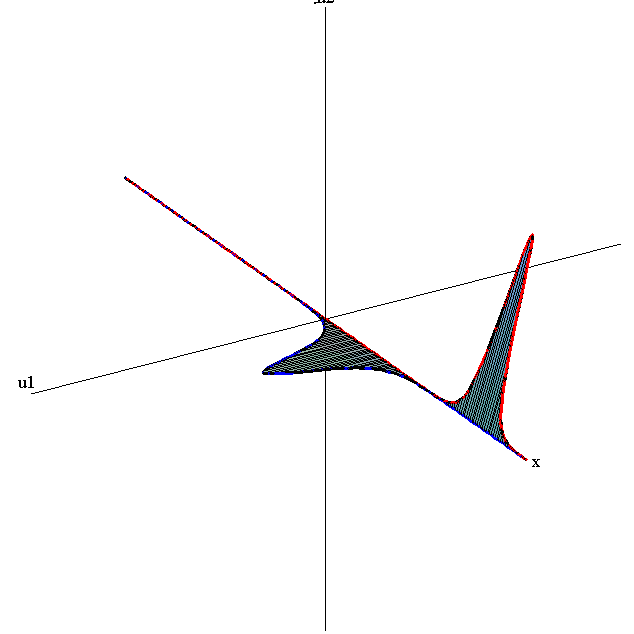speed ratio: c1/c2 = 3
relative orientation angle: Δφ = 0.6 π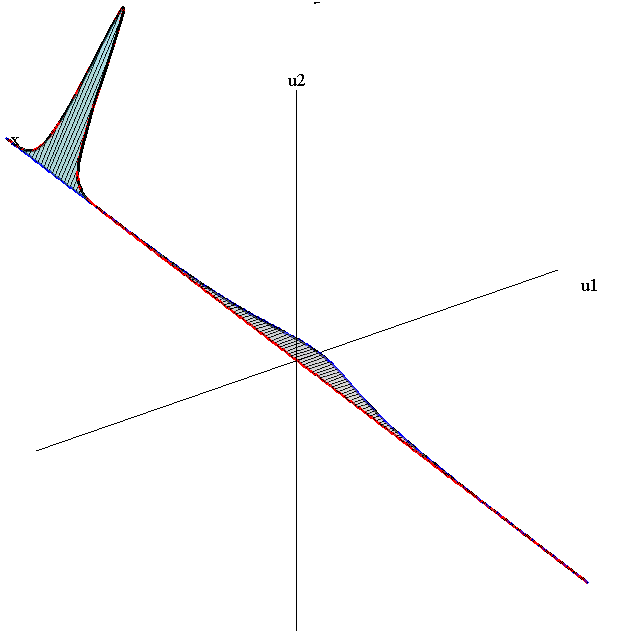speed ratio: c1/c2 = 50
relative orientation angle: Δφ = 0.35 π

#### Bounce-Exchange Interaction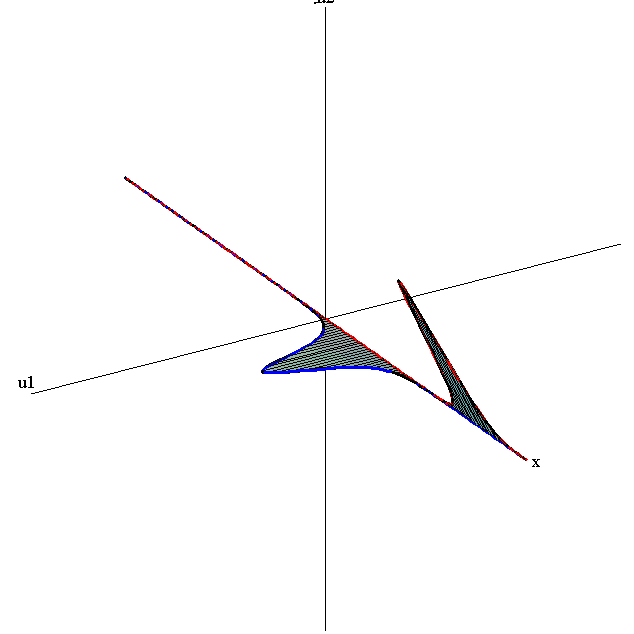speed ratio: c1/c2 = 3
relative orientation angle: Δφ = 0.35 π

#### Absorb-Emit interaction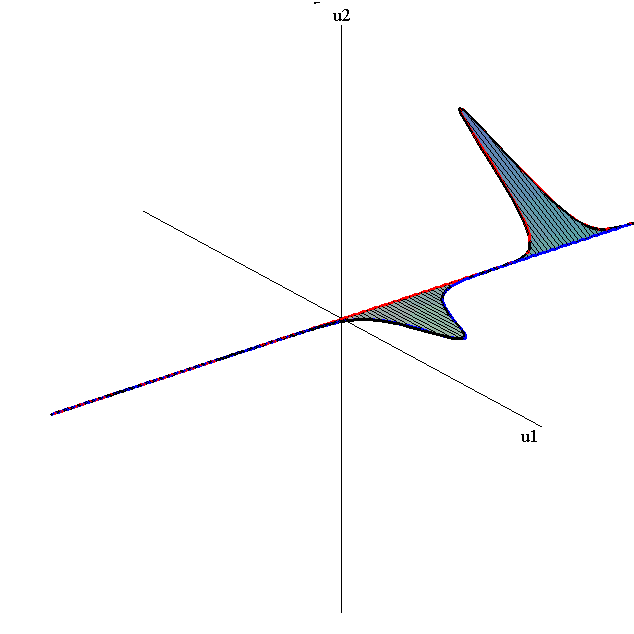speed ratio: c1/c2 = 3
relative orientation angle: Δφ = 0.85 π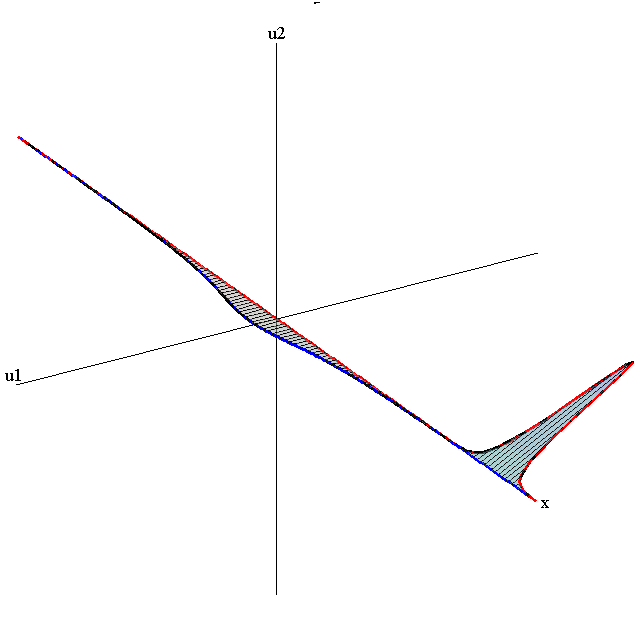speed ratio: c1/c2 = 50
relative orientation angle: Δφ = 0.85 π